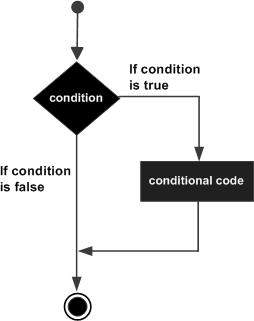# Python IF Statement

It is similar to that of other languages. The if statement contains a logical expression using which data is compared and a decision is made based on the result of the comparison.

## Syntax

```if expression:
statement(s)
```

If the boolean expression evaluates to TRUE, then the block of statement(s) inside the if statement is executed. If boolean expression evaluates to FALSE, then the first set of code after the end of the if statement(s) is executed.

## Flow Diagram### Example

```#!/usr/bin/python

var1 = 100
if var1:
print "1 - Got a true expression value"
print var1

var2 = 0
if var2:
print "2 - Got a true expression value"
print var2
print "Good bye!"
```

When the above code is executed, it produces the following result −

```1 - Got a true expression value
100
Good bye!
```
python_decision_making.htm